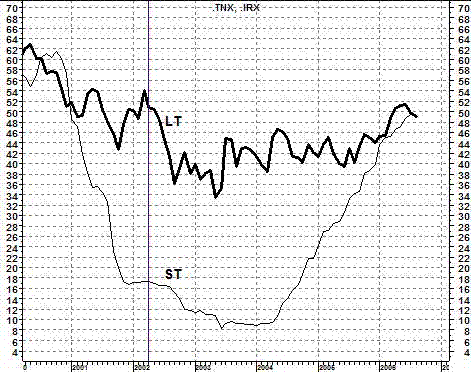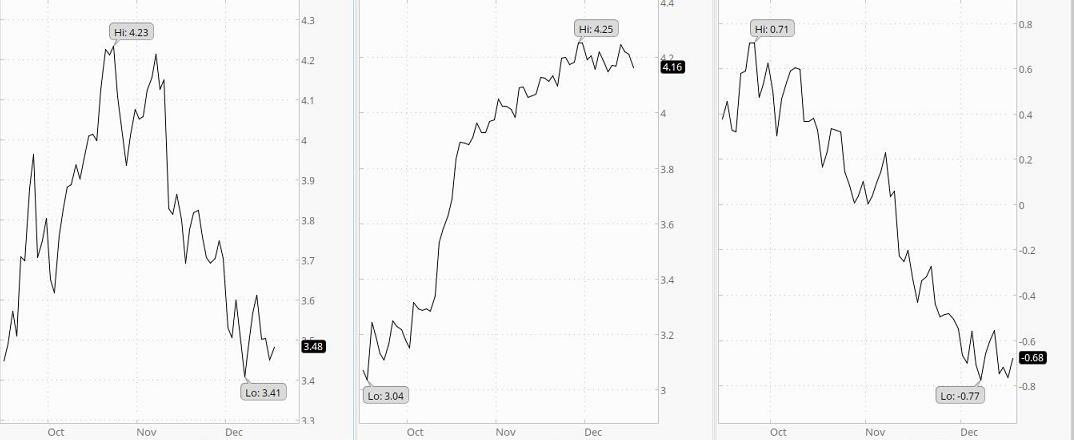Short-Term vs. Long-Term T-Rates

Relationship Between Short-term and Long-term Treasury
Rates: What the Interest Rate Spread Tells YouIn the chart, the light line (ST) traces the yield of short-term (13-week) treasuries and the dark line (LT) traces the yield of long-term (10-year) treasury bonds. Divide the numbers on the scale by ten to get the yields on these investments. For example, at the vertical line (4/30/02), LT rates were 50.9 ÷10 = 5.09% and ST rates were 17.3 ÷10 = 1.73% (ST rates were 3.36% less than LT rates). When ST rates are 1.3% to 2% below LT rates, investors expect normal economic growth (2-3 percent per year). When the spread is greater, they expect even faster growth because the Central Bank is likely pushing rates down. This reduces the cost of borrowing for companies so they can expand capital investment. When ST rates are higher than LT rates, Central Banks are probably trying to curb inflation. Economic conditions are expected to deteriorate. When ST rates exceed LT rates by 1.5% or more, there is a 70% chance the economy will go into recession within the next 12-months. The foregoing can serve as an investment guide. If ST rates are higher than LT rates, tighten stops or take other protective measures. If they are 1% or more higher, consider moving to cash (notice that the ST curve was this far above the LT curve in 2000 and that a 3-year bear market began in that year). When ST rates are less than 1% below LT rates, stable growth stocks are attractive. When ST rates are 1% to 3% less than LT rates, stocks are even more attractive (a stock-picker’s market). If the spread is more than 3%, invest with the premise that inflation could start to heat up soon. At the time show in the chart, the spread was -.034. ~ Dr. Felt

Expanded Discussion With Some Repeating
This was taken from another location on this Website (and at a different time ).  It covers the same information with different charts, with maybe a few other minor differences.

Interest Rates10-Year                             13-Week                             Spread